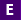#Not a Florida public school educator?

Access this resource
on CPALMS.com

### General Information

Subject(s): Mathematics, Science
Intended Audience: EducatorsSuggested Technology: Computer for Presenter, Computers for Students, Internet Connection, Adobe Acrobat Reader
Instructional Time: 40 Minute(s)
Keywords: Rounding, Rounding numbers, decimal place value, rounding decimal numbers, place value, tenths, hundredths, thousandths, decimal place value, decimal numbers, rounding decimal numbers, powers of 10, divide by 10, multiply by 10, expanded notation
Instructional Component Type(s):

## Aligned Standards

This vetted resource aligns to concepts or skills in these benchmarks.

## 1 Lesson Plan

Rounding Decimal Numbers - Lesson #3

This is the final lesson in the Rounding Decimal Numbers Unit and will bring together the mathematical concepts in lesson 1 and 2. This lesson then bridges the computer science coding and mathematical thought process together as students dissect code that rounds decimal numbers as well as building their own code to make rounding an automated process.

## Related Resources

Other vetted resources related to this resource.

## Lesson Plan

Rounding Decimal Numbers - Lesson #3:

This is the final lesson in the Rounding Decimal Numbers Unit and will bring together the mathematical concepts in lesson 1 and 2. This lesson then bridges the computer science coding and mathematical thought process together as students dissect code that rounds decimal numbers as well as building their own code to make rounding an automated process.

Type: Lesson Plan